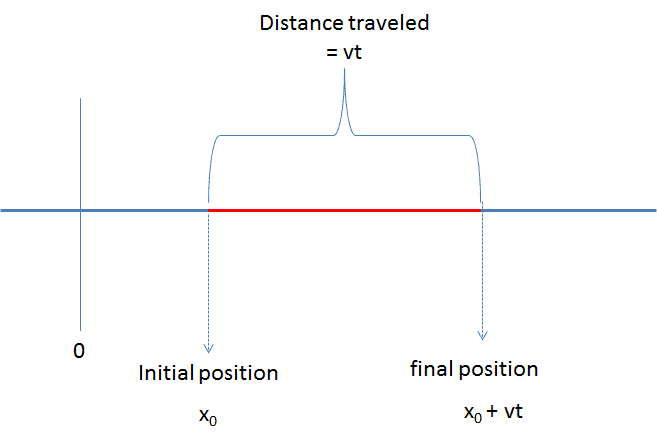# In the equation x = x₀ + vt, 'x₀' means what?

• B
• Indranil

#### Indranil

In the equation x = x₀ + vt, 'x₀' means what?

The subscript "0" pretty much always means the original/starting value.

The subscript "0" pretty much always means the original/starting value.
As I know x denotes 'displacement'. If x = 5 meters east, it means it's final position is 5 meters east but 'x₀' denotes what? is the object stationary in this case?

In the equation x = x₀ + vt, 'x₀' means what?

It means the initial position of an object.

As I know x denotes 'displacement'. If x = 5 meters east, it means it's final position is 5 meters east but 'x₀' denotes what? is the object stationary in this case?

If you write, ##x_0 = 5## then that would mean that the initial position of the object is, in a standard cartesian coordinate system (xy-plane), located at 5 units to the right of the origin.

As I know x denotes 'displacement'. If x = 5 meters east, it means it's final position is 5 meters east but 'x₀' denotes what? is the object stationary in this case?
If ##x_0 = 5##, this means that the object in question starts out at 5 units in the positive direction (usually eastwards or to the right) from the origin (where x=0). This is the initial position.

The ##x## value being spit out by the function gives you the final position of the object. This can be any new number.

However ##x## is not the displacement. The displacement would be ##\Delta x##, pronounced Delta ##x##. ##\Delta x## is the difference between ##x_0## and ##x##.

If ##x_0 = 5##, this means that the object in question starts out at 5 units in the positive direction (usually eastwards or to the right) from the origin (where x=0). This is the initial position.

The ##x## value being spit out by the function gives you the final position of the object. This can be any new number.

However ##x## is not the displacement. The displacement would be ##\Delta x##, pronounced Delta ##x##. ##\Delta x## is the difference between ##x_0## and ##x##.
Ok, then what would be the final position ( x ) from the concept above? what would be the final position value? I knew that the initial position is 0 and the final position is 5. Am I correct? Please explain.

Last edited:
In the equation x = x₀ + vt, 'x₀' means what?
x0 is the position at t = 0: the initial position.

x0 is the position at t = 0: the initial position.
Could you please provide me with a diagram so that I can clear my confusion? it's my humble request.

Could you please provide me with a diagram so that I can clear my confusion? it's my humble request.
I'm not sure what sort of diagram you're looking for. The equation ##x = x_0 + vt## applies to constant velocity along a single axis (in this case the x axis). It gives you the final position along that axis after some time "t" passes. The final position depends on where you started (given by x0) and the distance you traveled in that time (given by vt).

I'm not sure what sort of diagram you're looking for. The equation ##x = x_0 + vt## applies to constant velocity along a single axis (in this case the x axis). It gives you the final position along that axis after some time "t" passes. The final position depends on where you started (given by x0) and the distance you traveled in that time (given by vt).
Please provide me with any 'initial and final position' diagram. It would be very useful to me.

Please provide me with any 'initial and final position' diagram. It would be very useful to me.
Code:
x axis: -----0--------x0-----------------------xf----
^        ^                        ^
Origin   Start here               End here

•Jehannum#### Attachments

•Jehannum, lekh2003, russ_watters and 1 other person
In the equation x = x₀ + vt, 'x₀' means what?

These are the coordinate equations for rectilinear uniform motion. x₀ is the coordinate of the starting point of motion. And plus or minus shows the direction of motion. In one case, when plus, it moves toward plus infinity, and when minus, it moves toward minus infinity.
v- velocit
t-unit of time

Last edited by a moderator:
This thread is 5 years old and the OP is no longer at PhysicsForums. Your post does not add anything to what has been said before.